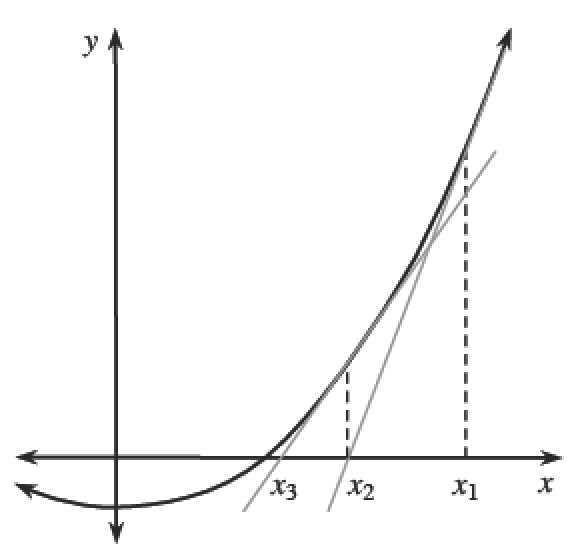### Home > CALC3RD > Chapter Ch7 > Lesson 7.3.5 > Problem7-159

7-159.

Use Newton’s Method to calculate the $x$-intercept of the function $y = x - 2 + \sin(x)$, correct to five decimal places.

A reasonable estimate is $x_1 = 1$.

$y(1)=1-2+\sin(1)\approx-0.1585$

$y'=1+\cos(x)$

$y'(1)=1+\cos(1)\approx 1.5403$

$x_2=1-\frac{-0.1585}{1.5403}\approx1.1029$

Newton's Method for Approximating Roots

Newton’s Method uses an iterative process to approximate a root ($x$-intercept) of a function.

$⇒$Begin with a point $x_{1}$ near the root. Write the equation of its tangent line at $x_{1}: y – f\left(x_{1}\right) = f ′\left(x_{1}\right)\left(x – x_{1}\right)$.

The $x$-intercept of this tangent line is $x_{2}$.
Solve for $x_{2}$ by setting $y = 0$.

$0 − f\left(x_{1}\right) = f ′\left(x_{1}\right)\left(x_{2} − x_{1}\right),$ so $x _ { 2 } = x _ { 1 } - \frac { f ( x _ { 1 } ) } { f ^ { \prime } ( x _ { 1 } ) }$.

$⇒$ To calculate $x_{3}$, solve for the root of the line tangent to $f$ at $x_{2}$. This process continues until the desired accuracy is met.

Therefore, each new approximation, $x_{n+1}$, can be found using $x _ { n + 1 } = x _ { n } - \frac { f ( x _ { n } ) } { f ^ { \prime } ( x _ { n } ) }$. Newton’s Method was very important before calculators were invented and represents an excellent example of an iterative process. For most functions it works well, but it is always possible to find examples where it does not.